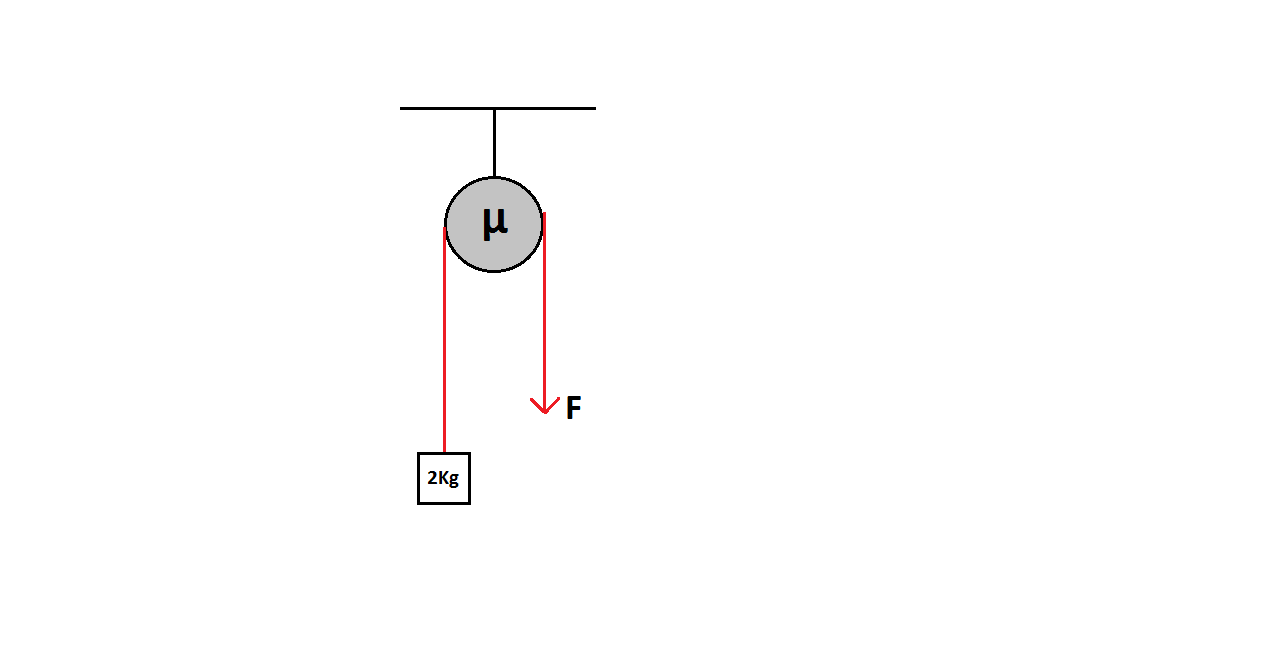# Pulley From The Other WorldA block of mass $2 \text{kg}$ is to be lifted with constant velocity by applying force $F$ down the rope that passes over a pulley having coefficient of friction $\mu$ w.r.t. the rope. If the pull required for this (in Newtons) is $X$, evaluate $\lfloor{X}\rfloor$.

Details and Assumptions
1) The pulley and rope are massless.
2) $\mu=2$
3) Take $g=10 ms^{-1}$.
4) The force is applied vertically downwards.
5) $\lfloor.\rfloor$ denotes the Greatest Integer Function.

×

Problem Loading...

Note Loading...

Set Loading...